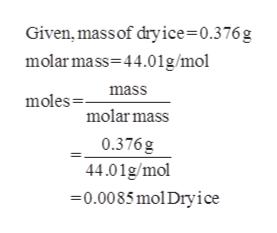# Calculate the number of moles present in a .376 g dry ice sample.Calculate the theoretical volume of the CO2 sample if it is sublimed at STP. Thanks so much whoever you are :)

Question
38 views

Calculate the number of moles present in a .376 g dry ice sample.

Calculate the theoretical volume of the CO2 sample if it is sublimed at STP.

Thanks so much whoever you are :)

check_circle

Step 1

Dry ice is the frozen carbon dioxide.

The number of moles present ...help_outlineImage TranscriptioncloseGiven, mass of dryice=0.376g molar mass 44.01g/mol mass moles molar mass 0.376g 44.01g/mol 0.0085 mol Dry ice fullscreen

### Want to see the full answer?

See Solution

#### Want to see this answer and more?

Solutions are written by subject experts who are available 24/7. Questions are typically answered within 1 hour.*

See Solution
*Response times may vary by subject and question.
Tagged in

### Chemistry